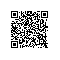# Python 中的 sys.argv 是个什么鬼？sys.argv[] 说白了就是一个从程序外部获取参数的桥梁 ，我们从外部取得的参数可以是多个 ，所以获得的是一个列表（list)，也就是说sys.argv其实可以看作是一个列表 ，所以才能用[]提取其中的元素 。其第一个元素（sys.argv）是程序本身 ，随后才依次是外部给予的参数 。

1. 本质是一个列表

import sys
print(type(sys.argv))
print(len(sys.argv))


C:\Users\Jan\test2\tensorflow-face-detection>python test_argv.py 1 2 3 4
<class 'list'>
5

1. 列表元素来自外部输入
2. 外部输入从1开始
3. argv 表示本身代码文件路径

import sys

script,first,second,third = sys.argv

print ("The script is called:{%s}"% script)
print ("The first variable is:{%s}"% first)
print ("The second variable is:{%s}"% second)
print ("The third variable is:{%s}"% third)


C:\Users\Jan\test2\tensorflow-face-detection>python test_argv.py 1 2 3
The script is called:{test_argv.py}
The first variable is:{1}
The second variable is:{2}
The third variable is:{3}


import sys
import os

os.system(sys.argv)使用钉钉扫一扫加入圈子
+ 订阅